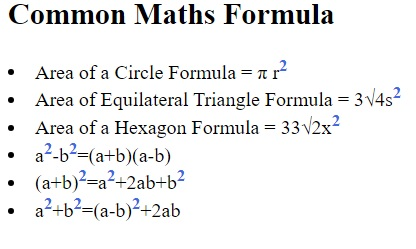# How to write superscript using HTML?

By IncludeHelp Last updated : November 15, 2023

## What is superscript?

Superscript is that text/number that is written above and on the right side of any character/word/digit. We use the superscript concept in almost every branch for example, in chemistry/biology we use superscript while writing equations, in math we use this concept while specifying the powers.

• DE = (Dm)2
• A2+B2=2AB
• H2l

Now what if we have some webpage where we post blogs related to chemistry subjects, in this case, what should we do if we have to use some superscript concept in our webpage?

In HTML, if we want to apply superscript to any text we use <sup> tag. Any text/digit you want to keep as superscript is written between these tags.

## Syntax

The syntax to create subscript is:

```<sup>text</sup>
```

## Example

In this example, we are displaying some common maths formulas on the webpage using the subscript tags.

```<!DOCTYPE html>
<html lang="en">
<meta charset="UTF-8">
<title>Title</title>
<!--
css style to set color and weight
for superscript -->
<style class="text/css">
sup{
color:royalblue;
font-weight: bolder;
}
</style>
<body>
<div>
<h2>Common Maths Formula</h2>
<li>Area of a Circle Formula = π r<sup>2</sup></li>
<li>Area of Equilateral Triangle Formula = 3√4s<sup>2</sup></li>
<li>Area of a Hexagon Formula = 33√2x<sup>2</sup></li>
<li>a<sup>2</sup>-b<sup>2</sup>=(a+b)(a-b)</li>
<li>(a+b)<sup>2</sup>=a<sup>2</sup>+2ab+b<sup>2</sup></li>
<li>a<sup>2</sup>+b<sup>2</sup>=(a-b)<sup>2</sup>+2ab</li>
</div>
</body>
</html>
```

We have simply applied the entire superscript digit within the <sup> tag We've also applied some CSS to show that we can apply CSS to this tag by just using the tag name as a selector.

## Output

The output of the above program is: